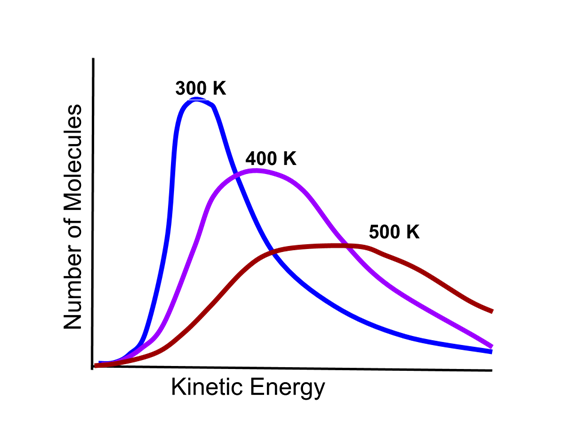# Enduring Understanding 5.A.1: Temperature

• Temperature refers to a measure of the average kinetic energy of atoms and molecules.
• All atoms/molecules in a sample are always in motion.
• Temperature is often expressed in Kelvin, K.
• Zero Kelvin (absolute zero) represents the temperature where there is no molecular motion/kinetic energy.
• When the average kinetic energy of particles in a sample doubles, the temperature in Kelvin doubles.
• The kinetic energy distribution of gas particles depends on the temperature of the gas sample, as illustrated in this Maxwell-Boltzmann distribution.
•• As the graphic indicates, the higher the temperature, the higher the average kinetic energy and more disperse the kinetic energy distribution becomes.
• The average velocity of gas particles depends on the temperature and the mass of the particles. Therefore, at the same temperature, a heavier gas will have a slower average velocity.
• Sample question 1: Samples of Helium, neon, and argon are mixed in a rigid container at 27 °C. Which gas (if any) will have the higher average particle velocity?
• Helium. The three gases will have the same average kinetic energy, since they are at the same temperature. Helium atoms have a lower mass than neon or argon, so their average velocity will be higher.
• Sample question 2: Which of the following temperature changes describes a doubling of average kinetic energy?
• 100 °C → 200 °C
• 200 °F → 400 °F
• 100 K → 200 K
• Answer: 100 K → 200 K. Temperature in kelvin is proportional to average kinetic energy.

 Related Links: Chemistry Chemistry Quizzes AP Chemistry Notes Catalysis

To link to this Temperature page, copy the following code to your site: